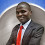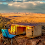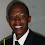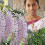# Excel Formula : Convert data from long to wide format

Suppose you have data that is stored in long format in excel. You want to reshape it to wide format.

The data is shown in the image below : -Reshape data from long to wide format

Step I : To pull unique values from Column A

Type the following formula in cell D2
=IFERROR(INDEX(\$A\$2:\$A\$100, MATCH(0,COUNTIF(\$D1:D\$1, \$A\$2:\$A\$100), 0)),"")
Press CTRL + SHIFT + ENTER to confirm this formula as it's an array formula. If this formula is entered correctly, you would see the formula inside the curly brackets {}.

Column A does not necessarily to be in numeric format. Similarly, Column B can take both numeric or character values.

Step II : Reshape data from long to wide format

Type the following formula in cell E2

=IF(COLUMN(A1)<=COUNTIF(\$A\$1:\$A\$100,"="&\$D2),OFFSET(INDIRECT("\$B"&MATCH(\$D2,\$A\$1:\$A\$100,0)),COLUMN(A1)-1,0),"")

Paste the formula to the right and down so that transformation happens correctly. You can use CTRL + R shortcut key to paste the formula to the right and CTRL + D shortcut key to paste the formula down.

Related Posts
ShareDeepanshu founded ListenData with a simple objective - Make analytics easy to understand and follow. He has over 10 years of experience in data science. During his tenure, he worked with global clients in various domains like Banking, Insurance, Private Equity, Telecom and HR.

13 Responses to "Excel Formula : Convert data from long to wide format"
1.The data in Column A must be numbers?
I tried text data and got wrong results

1.yes, it must definitely be numbers, in order

2.We have another formula for extracting unique numbers

Alternatively you can copy on a different sheet and drop duplicates

2.The second COUNTIF should be a MATCH() instead. It should be:

=IF(COLUMN(A1) <=COUNTIF(\$A\$1:\$A\$100,"="&\$D2),OFFSET(INDIRECT("\$B"& MATCH(\$D2,\$A\$1:\$A\$100,0)),COLUMN(A1)-1,0),"")

1.Thank you so much it works

3.Not so useful IMHO because the resulting columns have the various stocks listed helter-skelter, for instance, column E has Stocks D, O, D, Q, P. Usually you'd want all the D's stacked, etc.

4.Wow, cool post. I’d like to write like this too – taking time and real hard work to make a great article…

5.What if the table had more than two columns?

6.I have an excel sheet with four columns and more than 6000 rows. I have tried this formula and it only works for two columns and up to cell 100 across the two columns. How do I modify to suit my data?

7.Joseph Szymczak-ParkerJune 11, 2021 at 12:01 AM

Use the =Transpose(range) function.

8.You made some really good points there. I checked on the web to find out more about the issue and found most individuals will go along with your views on this site.

9.This is really useful. Can you please expain each formula. This will be helpful in applying this formula to larger data sheets.

10.Thanks! This is a good way to solve those problems ;). I would like to understand how works every formula in the same post, however, Great article!! 10/10

Next → ← Prev